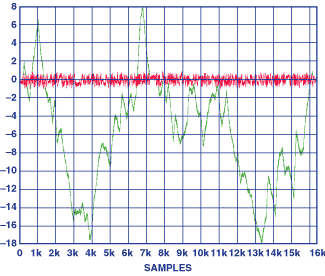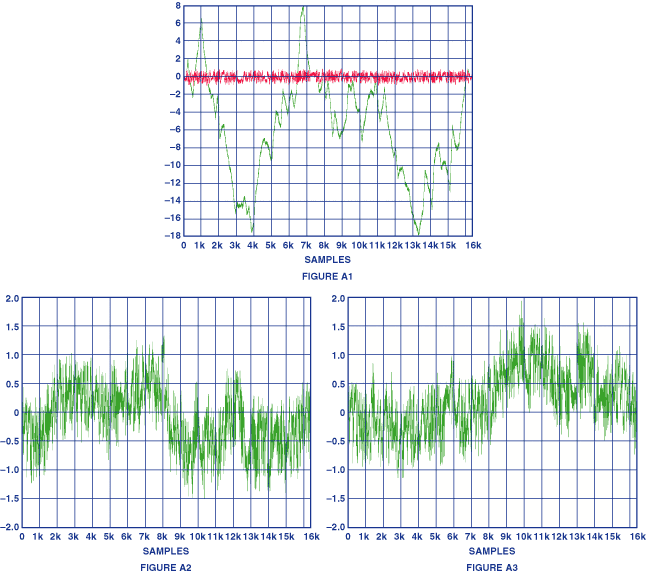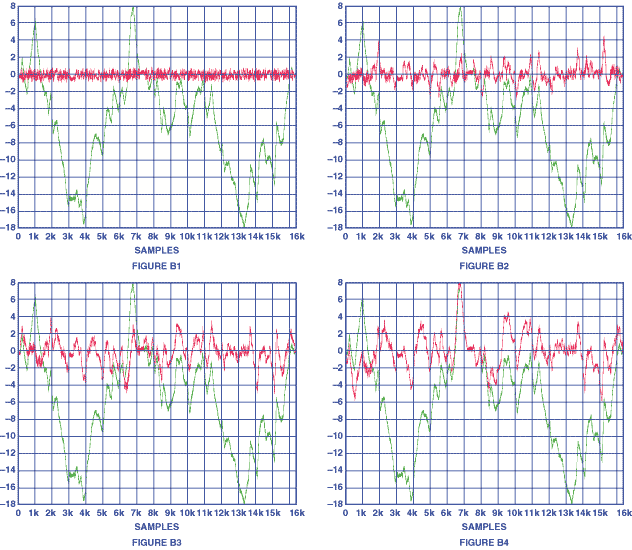# Getting 14-Bit Performance from a 32-Channel 14-Bit String DAC

### Overview

The AD5532 32-channel, 14-bit voltage output D/A converter can be used in DAC mode (used for accessing multiple analog representations of digital data) or Infinite Sample-and-Hold (ISHA) mode (for storing and accessing analog representations of analog data). The DACs have 14-bit monotonicity, but only ±0.39% integral nonlinearity. This article shows how the DACs can be calibrated to provide 14-bit performance.

In DAC mode, the selected DAC register is written to via the 3‑wire serial interface; the analog output (VOUT) for this DAC is then updated to reflect the new contents of the DAC register. DAC selection is accomplished via five address bits, A0‑A4. The reference, the voltage applied the OFFS_IN pin, and the gain of the output amplifier combine to determine the output range of the AD5532.

In ISHA mode, the input voltage, VIN, is sampled and converted into a digital word. The noninverting input to the selected (nth) output buffer (gain and offset stage) is tied to VIN during the acquisition period to avoid transient spurious outputs while the nth DAC acquires the correct code, a step completed in 16 µs max. The updated DAC output then is connected to the noninverting input of the nth output buffer and assumes control of its output voltage. Since the channel output voltage is effectively the output of a DAC with a fixed input, there is no droop associated with it. As long as power is maintained to the device, the output voltage will remain constant until this channel is addressed again.

The analog output is restricted to a range from VSS + 2 V to VDD – 2 V because of headroom constraints in the output amplifier. The device is operated with AVCC = 5 V ±5%, DVCC = 2.7 V to 5.25 V, VSS = –4.75 V to –16.5 V, and VDD = 8 V to 16.5 V; and it requires a stable +3‑V reference on REF_IN, as well as an offset voltage on OFFS_IN.

In DAC mode of operation, the AD5532’s DACs are guaranteed monotonic to 14-bits (differential nonlinearity <1 LSB)—thus ideally suiting them for closed-loop control applications. Accuracy, however, is limited by the space-saving string-DAC architecture. The DACs’ specified integral nonlinearity (INL) error is 0.39% max of full scale (0.15% typical), or 64 (24.5 typical) least-significant bits in a 14-bit device. We can thus say that worst-case DAC integral linearity is comparable to that of an 8-bit device, even though it has 14-bit resolution.

This level of worst-case performance is acceptable for many applications, especially considering that the AD5532 can at any time economically and compactly store and read out 32 analog data points with 61-part-per-million resolution. But there are many applications where, although this kind of performance is essential, better accuracy is also necessary. Our purpose here is to show a way to calibrate the AD5532 for full 14-bit performance with a maximum of only 256 calibration coefficients (128 data points) per DAC, using a controller and a maximum of 8,192 slots of memory. Figure 2 shows the kind of improvement that can be obtained.Figure 2. Un-calibrated linearity error compared with post-calibration linearity error for a 128-point calibration of a typical AD5532 channel at 25°C.

The following describes the basic DAC architecture and a method of calibration that can be easily implemented to achieve an INL error level of 1 LSB.

### DAC Architecture

The common string DAC is one of the oldest and simplest DAC circuit concepts. Resistor-string DAC implementations are inherently monotonic by design and are characterized by simplicity, small size (per resistor) and low power consumption. But a major drawback is that 2N resistors are required to implement it directly—e.g., 16,384 for 14 bits. In order to reduce the number of resistors and die size, the AD5532 incorporates two 128-resistor strings (7 bits)—a main string DAC for the 7 more-significant bits, and a 7-bit sub-string DAC. The basic architecture is shown in Figure 3 (US patent 5,969,657). The sub string DAC straddles up and down the main string, always in parallel with one of the main string resistors.

Directly multiplying potentiometer-style resistive DACs suffer nonlinearity of the step size due to the variable loading of the sub string in parallel with the main string. But in DACs such as the AD5532, the loading of the substring is the same at all levels and is treated, not as a major error source, but as a characteristic of the DAC transfer function. The sub string loading error is 1 LSB.

The AD5532 DAC, using the architecture outlined above, is made up of a 7-bit-string main DAC (128 resistors) and a 7-bit-string sub-DAC (127 resistors) that bridges individual resistors of the main DAC. The integral nonlinearity error (INL) is determined by the matching of the main-DAC resistors. The sub-DAC provides the lower 127 codes of the transfer function. The linearity of the sub-DAC can be approximated by piecewise-linear segments.

### DAC Transfer Function:

The main DACs on the AD5532 are lifted off DACGND by typically 50 mV (by means of resistors at the bottom of the DAC). So the bottom of a DAC is typically at 50 mV, while the top of the DAC is typically at Vref. Figure 4 shows how the nominal DAC transfer function is derived for a single channel.

The standard DAC transfer function that applies to the AD5532 is:

where:

N = DAC code value in decimal (0<N<16383)

Vref_top= Vref and Vref_bottom= 50 mV (typ)

The output stage then amplifies and offsets the Vdac output as follows:

where:

Gain is typically 3.52 and Voffs_In is whatever the user programs.

For Voffs_In = 0 and Vref = 3 V

Vout (zero code) = 3.52 * 50mV = 176 mV (typ)

Vout (mid-scale)= 3.52 *1.525V = 5.368 V (typ)

Vout (full-scale)= 3.52 * 3V = 10.56V (typ)

### Calibration Scheme:

As noted above, this calibration scheme applies to all parts in the AD5532 family. The overall INL curve can be thought of as 128 piecewise-linear segments—corresponding to deviations in resistance value in the upper string—which are then interpolated linearly in the lower string. Because the small resistance deviations in the upper resistor string—which produce significant nonlinearities at the 14-bit level—will vary from channel-to-channel, and part-to-part, there is no “typical” INL curve; each DAC needs to be individually calibrated. The calibration scheme outlined here generates corrections to the lower 128 codes using an Mx + C approximation for correction values in each segment. C is the required correction at the beginning of a segment, M is the stored slope to the beginning of the next segment, and x is the analog ratio corresponding to a given 7-bit code.

Thus the user can develop a calibration table by measuring the difference, C, between the expected value and the actual value at each of the upper 128 codes, calculating the incremental slopes (M), and storing both values in memory for every 128 point interval, as shown in Figure 5. Then, during run-time, determine the segment, and thus C & M, from the upper 7 bits, compute the interpolation value determined by the lower 7 bits, and apply the correction to the DAC input.

Calibrating every 128 codes i.e., every segment, will reduce the INL error to less than ±1 LSB at the 14-bit level from the worst case ±64 LSBs for the uncalibrated DAC. If all the correction data must be stored in less memory than 8192 words, the number of calibration points can be reduced by increasing the calibration interval to 256 or 512 points—but this will reduce the overall integral linearity.

Figure 6 is a graph of linearity error for an AD5532 DAC channel before calibration, typically of the order of 10-bits. In all these plots, the Y-axis represents the linearity error expressed in LSBs (1 LSB = 61 ppm), while the X-axis is the 14-bit code loaded to the DAC.

Figure 7 shows the nonlinearity errors on the same channel, following the implementation of a 128-point calibration, as outlined above. It can be seen that the INL error is now within ±1 LSB.

The plots in figures 6 and 7 are at 25°C. Appendix A shows the linearity errors at –40°C and +85°C following the implementation of a 128-point calibration scheme at 25°C. The worst-case errors appear to be about twice as great as at 25°C.

As noted above, calibration can also be implemented using a smaller number of calibration points. The increase in linearity errors that results from using fewer calibration points is demonstrated in Appendix B.

### Hardware Implementation

Figure 8 shows a typical hardware implementation using the AD5532. Generally the controller writes directly to the AD5532, providing addressing and calculating calibrated data input values to update the relevant channels.

The calibration scheme requires the addition of a memory block to store the M and C calibration data for each segment in the DAC transfer function. Using a 128-point calibration scheme, 256 calibration coefficients need to be stored for each DAC.

Calibrating the complete AD5532 requires that 8192 coefficients be stored. In terms of memory size, the slope coefficient (M) will typically require 6 bits and the offset coefficient (C) also requires about 6 bits. The memory size required can be reduced at the expense of accuracy as noted above and in Appendix B.

In writing data to a specific DAC, the controller takes the input code and goes to the memory to pick up the relevant M and C coefficients for the segment defined by the input code. The controller then performs a linear interpolation to determine the correct code to write to the DAC.

### Conclusion

Using a simple interpolation scheme, it is possible to dramatically improve the linearity performance of the AD5532 family of DAC products.

We have shown that 14-bit linearity performance can be achieved following a 128-point calibration at 25°C. Pre-calibration linearity is typically at the 8-to-10-bit level.

All that is required to upgrade an existing AD5532 for improved performance in a system with computing power is the ability to generate calibration information and provide a memory block to store the calibration coefficients.

### Appendix A. Linearity at other temperatures after calibration at 25°C.

Figure A1 shows the un-calibrated linearity performance and the post-calibration linearity error following a 128-point calibration on a single AD5532 channel at 25°C. Figures A2 and A3 show the performance vs. temperature following the calibration at 25°C. The plots show the linearity error (Y axis) in LSBs vs. digital input code (x axis).Figure A1, 2, 3. AD5532 uncalibrated linearity errors and the improved linearity following a 128-point calibration at 25°C, –40°C, and 85°C. Note the change in scale for A2 and A3.

### Appendix B. Achievable performance with fewer calibration points

Optimum performance in calibrating the AD5532—with reasonable effort—is achieved by implementing a 128 point calibration scheme. In order to reduce the calibration time and the memory requirement, the number of calibration points can be reduced at the expense of overall accuracy. The plots included in Figures B1,2,3,4 compare the pre-calibration errors with the successively reduced improvement achieved using 128, 64, 32, and 16 calibration points (25°C).Figures B1, 2, 3, 4. AD5532 linearity performance without calibration—and following respective 128-, 64-, 32-, and 16-point calibrations at 25°C.

### 謝辞

We wish to thank Donal Geraghty, Patrick Kirby, John O'Sullivan and Catherine Redmond for their valuable contributions.